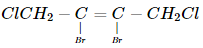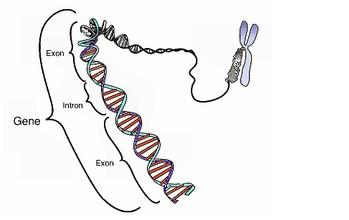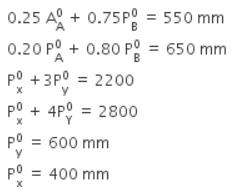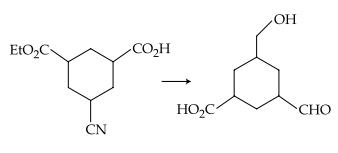Courses

# JEE(MAIN) Chemistry Mock Test - 3

## 30 Questions MCQ Test JEE Main Mock Test Series 2020 & Previous Year Papers | JEE(MAIN) Chemistry Mock Test - 3

Description
This mock test of JEE(MAIN) Chemistry Mock Test - 3 for JEE helps you for every JEE entrance exam. This contains 30 Multiple Choice Questions for JEE JEE(MAIN) Chemistry Mock Test - 3 (mcq) to study with solutions a complete question bank. The solved questions answers in this JEE(MAIN) Chemistry Mock Test - 3 quiz give you a good mix of easy questions and tough questions. JEE students definitely take this JEE(MAIN) Chemistry Mock Test - 3 exercise for a better result in the exam. You can find other JEE(MAIN) Chemistry Mock Test - 3 extra questions, long questions & short questions for JEE on EduRev as well by searching above.
QUESTION: 1

Solution:
QUESTION: 2

Solution:
QUESTION: 3

### The spectrum produced due to transition of an electron from M to L shell is

Solution:
QUESTION: 4

The correct order of increasing energy of atomic orbitals is

Solution:
QUESTION: 5

Combination of two AO's lead to the formation of

Solution: According to Linear Combination Of Atomic Orbitals(LCAO), the combination of 2 AO', give 2 MO's one of which is bonding MO while the other is antibonding MO.
QUESTION: 6

Elimination of bromine from 2- bromobutane results in the formation of

Solution:
QUESTION: 7

IUPAC name of CH₃-O-C₂H₅ is

Solution:
QUESTION: 8

The name ofaccording to IUPAC nomenclature system is

Solution:
QUESTION: 9

The bond order in NO is 2.5 while that in NO+ is 3. Which of the following statements is true for these two species?

Solution:

Higher the bond order, shorter will be the bond length. Thus, NO+ is having higher bond order than that of NO so NO+ has shorter bond length.

QUESTION: 10

The segment of DNA which acts as the instructional manual for the synthesis of the protein is

Solution:

A gene is the molecular unit of heredity of a living organism. It is widely accepted by the scientific community as a name given to some stretches ofdeoxyribonucleic acids (DNA) and ribonucleic acids (RNA) that code for apolypeptide or for an RNA chain that has a function in the organism, though there still are controversies about what plays the role of the genetic materialQUESTION: 11

K₄[Fe(CN)₆] is a

Solution:
QUESTION: 12

What are the products formed in the reaction of xenon hexafluoride with silicon dioxide

Solution:
QUESTION: 13

The hardness of transition elements is due to their

Solution:

Transition elements show metallic character as they have low ionization energies and have several vacant orbitals in their outermost shell. This property favors the formation of metallic bonds in the transition metals and so they exhibit typical metallic properties.These metals are hard which indicates the presence of covalent bonds. This happens because transition metals have unpaired d-electrons. The d-orbital which contains the unpaired electrons may overlap and form covalent bonds. Higher the number of unpaired electrons present in the transition metals, more is the number of covalent bonds formed by them. This further increases the hardness of the metal and its strength.

QUESTION: 14

Sodium nitroprusside reacts with sulphide ion to give a purple colour due to the formation of :

Solution:
QUESTION: 15

To a solution containing equimolar mixture of sodium acetate and acetic acid, some more amount of sodium acetate solution is added. The pH of mixture solution

Solution:
QUESTION: 16

The reason for geometrical isomerism by 2-butene is

Solution:
QUESTION: 17

If chloroform is left open in air in presence of sun rays

Solution:
QUESTION: 18

Ionisation potential of hydrogen is

Solution:

Hydrogen has higher ionisation potential than chlorine, because hydrogen has only one electron and small size. This electron experiences the direct attraction of the nucleus and there is no shielding effect. Whereas in Cl the combined effect of the increase in the atomic size and the screening effect more than compensates the effect of the increased nuclear charge. Consequently, the valence electrons become less firmly held by the nucleus and ionisation potential decreases.

QUESTION: 19

Which is an example of thermosetting polymer?

Solution:
QUESTION: 20

KO2 (potassium super oxide) is used in oxygen cylinders in space and submarines because it

Solution:

Because it absorbs CO2 and increases O2 concentration according to the following reaction

4KO2 + 2CO2 → 2K2CO3 + 3O2

QUESTION: 21

Benzene and toluene form nearly ideal solutions. At 20oC, vapour pressure of benzene is 75 torr and that of toluene is 22 torr. The partial vapour pressure of benzene at 20oC for a solution containing 78 g of benzene and 46 g of toluene is ___ torr.

Solution: Find moles of benzene & toluene:
Nb=1 ; Nt=1/2
total moles=1+1/2=3/2
Partial pressure=mole fraction*pressure of pure liq
PP of benzene=[Nb/(total moles)]*pressure of benzene
=1/(3/2)*75=50torr
QUESTION: 22

On heating an aliphatic primary amine with chloroform and ethanolic potassium hydroxide, the organic compound formed is

Solution:
QUESTION: 23

Which is used for ending charge on colloidal solution?

Solution:
QUESTION: 24

Which one of the following ions exhibits colour in aqueous solution?

Solution:
QUESTION: 25

Diethyl amine when treated with nitrous acid yields

Solution:
QUESTION: 26

The Henry's law constant for the solubility of N2 gas in water at 298 K is 1.0 x 105 atm. The mole fraction of N2 in air is 0.8. The number of moles of N2 from air dissolved in 10 moles of water at 298 K and 5 atm pressure is

Solution:
QUESTION: 27
Two liquids X and Y form an ideal solution. At 300 K, vapour pressure of the solution containing 1 mol of X and 3 mol of Y is 550 mm Hg. At the same temperature, if 1 mol of Y is further added to this solution, vapour pressure of the solution increases by 10 mm Hg. Vapour pressure (in mm Hg) of X and Y in their pure states will be, respectively.
Solution:QUESTION: 28

The reagent(s) for the following conversion,Solution:
QUESTION: 29

Both [Ni(CO)4] and [Ni(CN)4]2- are diamagnetic. The hybridisations of nickel in these complexes, respectively, are

Solution:

In [Ni(CO)4​], Ni is in 0 oxidation state so there are 8 electrons in 3 d subshell and 2 electrons in 4s. CO is the strong ligand, so it does pairing with the d subshell atom leaving one d subshell with unpaired electron.now the electron from s shell shifts to d creating vacant space in s subshell and thus the hybridization is sp3

In [Ni(CN)4​]2−, Ni is in +2 oxidation state so it has 8 electrons in d subshell and CN is also a strong ligand which pairs with d subshell atom and leaves one d orbital empty and thus its hybridization is dsp2

QUESTION: 30

Among the alkenes which one produces tertiary butyl alcohol on acid hydration

Solution: